## UGC NET Dec-2020 and June-2021 Paper-2

 Question 1
Given below are two statements Statement I: LL(1) and LR are examples of Bottom up parsers. Statement II: Recursive descent parser and SLR are examples of Top down parsers In light of the above statements, choose the correct answer from the options given below
 A Both Statement I and Statement II are false B Both Statement I and Statement II are true C Statement I is false but Statement II is true D Statement I is true but Statement II is false
Question 1 Explanation:
LL(1) is a Non Recursive descent Top down parser. LR is Bottom up Parser.
Recursive Descent Parser is top down parser with backtracking and SLR is one of four LR-Parsers(LR(0),SLR(1),LALR(1),CLR(1)).
Since LR is bottom up parser so SLR is example of Bottom up parser.Question 2
Match List I with List II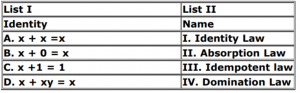Choose the correct answer from the options given below:
 A A - I, B -III , C - IV, D - II B A - III, B -I , C - II, D - IV C A - III, B -I , C - IV, D - II D A - III, B -IV , C - I, D - II
 Question 3
In software testing, beta testing is the testing performed by _______________.
 A potential customers at the developer's location B potential customers at their own locations C product developers at the customer's location D product developers at their own locations
Question 3 Explanation:
Explanation: Alpha Testing: The alpha test is conducted at the developer's site by a customer. The software is used in a natural setting with the developer "looking over the shoulder" of the user and recording errors and usage problems. Alpha tests are conducted in a controlled environment.
Beta Testing: The beta test is conducted at one or more customer sites by the end-user of the software. Unlike alpha testing, the developer is generally not present. Therefore, the beta test is a "live" application of the software in an environment that cannot be controlled by the developer.
 Question 4
Given below are two statements
Statement I: In an undirected graph, number of odd degree vertices is even.
Statement II: In an undirected graph, sum of degrees of all vertices is even.
In light of the above statements, choose the correct answer from the options given below.
 A Both Statement I and Statement II are false. B Both Statement I and Statement II are true. C Statement I is false but Statement II is true. D Statement I is true but Statement II is false.
Question 4 Explanation:
Both statements are true. First is true due to the handshaking lemma. And Second is true because we know sum of degrees can be given by formula 2*(Number of edges). Any number multiplied by 2 is always even.
 Question 5
Given the following STUDENT-COURSE scheme:
STUDENT(Rollno, Name, courseno)
COURSE (courseno, coursename, capacity),
where Rollno is the primary key of relation STUDENT and courseno is the primary key of relation COURSE. Attribute coursename of COURSE takes unique values only. Which of the following query(ies) will find total number of students enrolled in each course, along with its coursename.
A. SELECT coursename, count(*) 'total' from STUDENT natural join COURSE group by coursename;
B. SELECT C.coursename, count(*) 'total' from STUDENT S, COURSE C where S.courseno=C.courseno group by coursename;
C. SELECT coursename, count(*) 'total' from COURSE C where courseno in (SELECT courseno from STUDENT);
 A A and B only B A only C B only D C only
 Question 6
what language is accepted by the pushdown antomachineA L = { w | na(w) <= nb(w), w ε {a, b}+}} B L = { w | na(w) = nb(w), w ε {a, b}+}} C L = { w | nb(w) <= na(w), w ε {a, b}+}} D L= {wwR | w ε {a, b}+}
Question 6 Explanation:Question 7
For which value of n is Wheel graph Wn regular?
 A 2 B 3 C 4 D 5
 Question 8
Next five questions are based on the following passage.
Consider a domain consisting of three Boolean variables Toothache, Cavity, and Catch. The full joint distribution is a 2*2*2 table as shown in the figure below.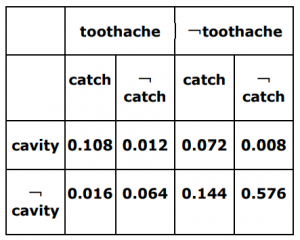The probability for Cavity, given that either Toothache or Catch is true, P(Cavity | toothache V catch) is _______.
 A 0.4615 B 0.5384 C 0.6 D 0.8
 Question 9
The order of a leaf node in a B+ tree is the maximum number of (value, data record pointer) pairs it can hold. Given that the block size is 1K bytes, data record pointer is 7 bytes long, the value field is 9 bytes long and a block pointer is 6 bytes long, what is the order of the leaf node?
 A 63 B 64 C 67 D 68
Question 9 Explanation:
Using the formula dp*m+vp*m+bp < =block size we get the order as 63. Here dp is Data
Pointer(7B), vp is value size(9B), bp is Block Pointer and m is the order of leaf.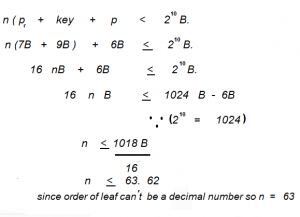Question 10
Given the following STUDENT-COURSE scheme:
STUDENT(Rollno, Name, Courseno)
COURSE(Courseno, Coursename, Capacity),
where Rollno is the primary key of relation STUDENT and Courseno is the primary key of relation COURSE. Attribute Coursename of COURSE takes unique values only. The number of records in COURSE and STUDENT tables are 3 and 5 respectively. Following relational algebra query is executed: R=STUDENT X COURSE
Match List I with List II in context to the above problem statement.Choose the correct answer from the options given below:
 A A -I , B -III , C -IV , D - II B A -I , B -III , C -IV , D - II C A -I , B -III, C -II , D - IV D A -III , B -I , C -IV , D - II
Question 10 Explanation:
Lets say STUDENT Relation has ‘p’ number of attributes and COURSE Relation has ‘q’ number of attributes.And lets say STUDENT Relation has 'm' records and COURSE Relation has ‘n’ tuples. And let's say 'R' is the result of Cross Product of STUDENT X COURSE.
Then Cardinality of R is m*n
And Degree of R is p+q. Foreign Key of relation STUDENT is Courseno and there is no foregin key in COURSE relation.
 Question 11
How many ways are there to assign 5 different jobs to 4 different employees if every employee is assigned at least 1 job?
 A 1024 B 20 C 240 D 625
 Question 12
Next five questions are based on the following passage. Consider a domain consisting of three Boolean variables Toothache, Cavity, and Catch. The full joint distribution is a 2*2*2 table as shown in the figure below.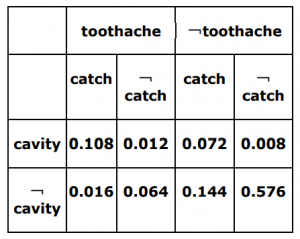The marginal probability of cavity P(cavity) is __________.
 A 0.08 B 0.12 C 0.2 D 0.216
 Question 13
Consider following two statements:
Statement I: Relational database schema represents the logical design of the database.
Statement II: Current snapshot of a relation only provides the degree of the relation.
In the context to the above statements, choose the correct option from the options given below:
 A Both Statement I and Statement II are FALSE B Both Statement I and Statement II are TRUE C Statement I is FALSE but Statement II is TRUE D Statement I is TRUE but Statement II is FALSE
Question 13 Explanation:
Relational databases schema were originally proposed to separate the physical storage of data from its conceptual representation and to provide a mathematical foundation for data representation and querying. It represents the logical design of the database. Hence statement 1 True.
Current snapshot or instance of a relation represent everything about the relation like degree of the relation, cardinality of the relation etc. Hence statement 2 is wrong.
 Question 14
Which of the graphs is/are planar?choose the correct answer from the options given below:
 A A and B only B A only C B and C only D B only
Question 14 Explanation:
A planar graph is a graph that can be embedded in the plane, i.e., it can be drawn on the plane in such a way that its edges intersect only at their endpoints.
In other words, it can be drawn in such a way that no edges cross each other.
Option-A is planar.
Option-B is planarOption-C is non planar
 Question 15
A data structure is required for storing a set of integers such that each of the following operations can be done in O(log n) time, where n is the number of elements in the set. Deletion of the smallest element
Insertion of an element if it is not already present in the set
Which of the following data structures can be used for this purpose?
 A A balanced binary search tree can be used but not a heap. B A heap can be used but not a balanced binary search tree. C Both balanced binary search tree and heap can be used. D Neither balanced binary search tree nor heap can be used.
Question 15 Explanation:
A Balanced Binary Tree is nothing but an AVL Tree. And Time complexity of an AVL tree for finding an element is (Log n) in the worst case. Heaps are good only for min/max elements. Min heap will have minimum element on root and max element will have maximum at root. But to insert an element which may or may not be present in the heap will take O (N) in the worst case because we need to check all the elements of the heap.
 Question 16
In software engineering, what kind of notation do formal methods predominantly use?
 A Computer code B Diagrammatic C Mathematical D Textual
Question 16 Explanation:
Explanation: Formal methods used in developing computer systems are mathematically based techniques for describing system properties. Such formal methods provide frameworks within which people can specify, develop, and verify systems in a systematic, rather than ad hoc manner.
A method is formal if it has a sound mathematical basis, typically given by a formal specification language. This basis provides a means of precisely defining notions like consistency and completeness, and more relevantly, specification, implementation and correctness.
 Question 17
The V components in MVC are responsible for:
 A Business logic and domain objects. B Security of the system. C Translating between user interface actions/events and operations on the domain objects. D User interface.
Question 17 Explanation:
MVC (Model-View-Controller) is a pattern in software design commonly used to implement user interfaces, data, and controlling logic. It emphasizes a separation between the software's business logic and display. This "separation of concerns" provides for a better division of labor and improved maintenance.
The three parts of the MVC software-design pattern can be described as follows:
Model: Manages data and business logic.
View: Handles layout and display.
Controller: Routes commands to the model and view parts.
 Question 18
Identify the correct order of the following five levels of Capability Maturity Model (from lower to higher) to measure the maturity of an organization's software process.
A. Defined
B. Optimizing
C. Initial
D. Managed
E. Repeatable
Choose the correct answer from the options given below
 A C, A, E, D, B B C, B, D, E, A C C, E, A, B, D D C, E, A, D, B
Question 18 Explanation:Question 19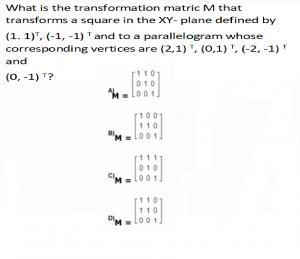A A B B C C D D
 Question 20
Given below are two statements
Statement I: Breadth-First Search is optimal when all the step costs are equal whereas uniform-cost search is optimal with any step-cost.
Statement II: When all the step costs are same uniform-cost search expends more nodes at depth d than the Breadth-First Search.
In light of the above statements, choose the correct answer from the options given below
 A Both Statement I and Statement II are false B Both Statement I and Statement II are true C Statement I is false but Statement II is true D Statement I is true but Statement II is false
 Question 21
Next five questions are based on the following passage.
Consider a domain consisting of three Boolean variables Toothache, Cavity, and Catch. The full joint distribution is a 2*2*2 table as shown in the figure below.P(cavity V toothache) is __________.
 A 0.12 B 0.2 C 0.28 D 0.6
 Question 22
Given a relation scheme R(x,y,z,w) with functional dependencies set F={x- > y, z- > w}. All attributes take single and atomic values only.
A. Relation R is in First Normal FORM
B. Relation R is in Second Normal FORM
C. Primary key of R is xz
Choose the correct answer from the options given below:
 A A and C only B B and C only C B only D C only
Question 22 Explanation:
The Primary Key is xz.
There is Partial dependency from x - > y and z - > w. It is Given that all attributes take single and atomic values only.
So the relation is in 1NF but not in 2NF and the key is xz.
 Question 23
A system has 99.99% uptime and has a mean-time-between-failure of 1 day. How fast does the system have to repair itself in order to reach this availability goal?
 A 10 Seconds B 11 Seconds C 12 Seconds D 9 Seconds
 Question 24
Which of the following are logically equivalent?
A.¬ p →(q → r) and q →(p v r )
B. ( p →q)→r and p →(q →r)
C. (p →q )→( r → s) and (p → r)→(q → s)
Choose the correct answer from the options given below:
 A A and B only B A and C only C A only D B and C only
Question 24 Explanation: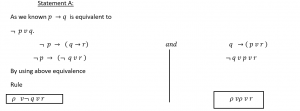Question 25
Consider the following 3 processes with the length of the CPU burst time given in milliseconds: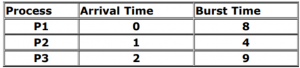What is the average waiting time for these processes if they are scheduled using the preemptive shortest job first scheduling algorithm?
 A 2.66 B 4.66 C 5.5 D 6
Question 25 Explanation: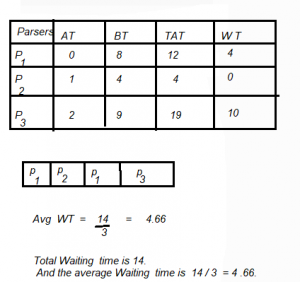There are 25 questions to complete.

Register Now